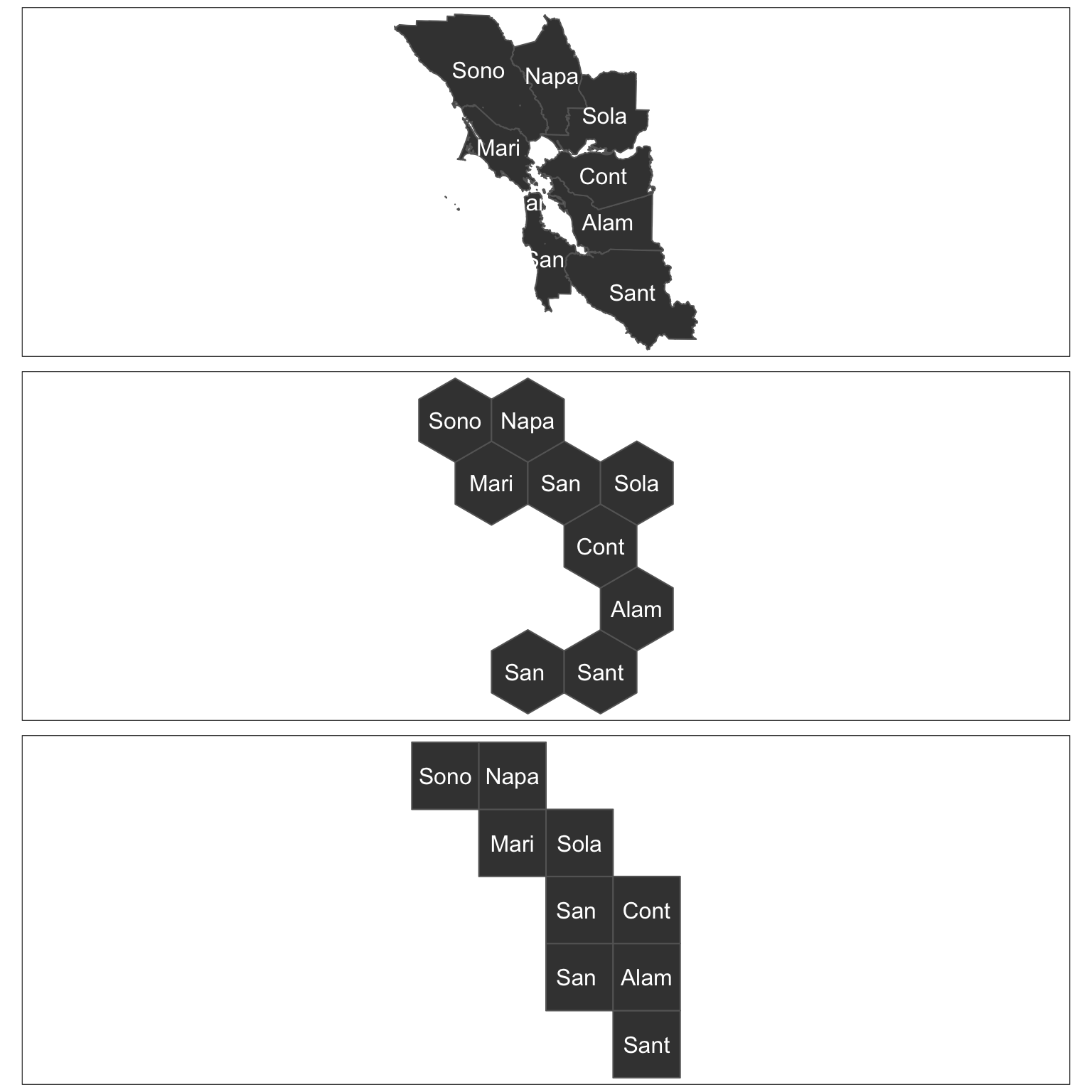# Algorithmic tesselation with geogrid

Joseph Bailey 2018-12-07

# geogrid

Turn geospatial polygons like states, counties or local authorities into regular or hexagonal grids automatically.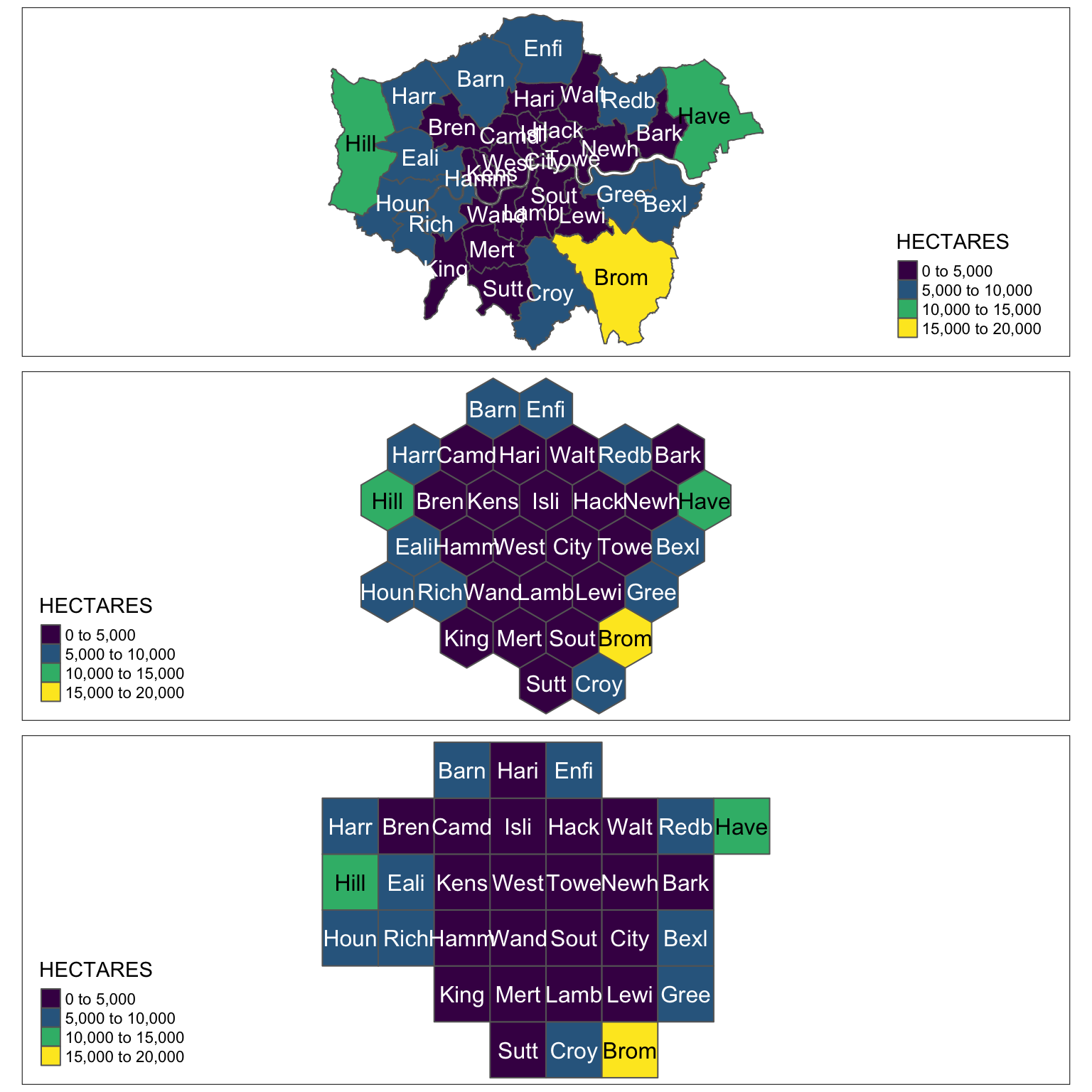## Intro

Using geospatial polygons to portray geographical information can be a challenge when polygons are of different sizes. For example, it can be difficult to ensure that larger polygons do not skew how readers retain or absorb information. As a result, many opt to generate maps that use consistent shapes (i.e. regular grids) to ensure that no specific geography is emphasised unfairly. Generally there are four reasons that one might transform geospatial polygons to a different grid (or geospatial representation):

1. We may use cartograms to represent the number of people (or any value) within a particular geography. For more information and examples see here and here. This cartogram approach changes the size of a particular geography in-line with the values that one seeks to visualise.
2. We may use grids to bin data and typically visualise the spatial density of a particular variable. For an example see here.
3. We may use grids to segment a geographical region. For example tesselation can be used in biological sampling or even in generating game environments.
4. We may use grids to ‘fairly’ represent existing geographical entities (such as US states, UK local authorities, or even countries in Europe). For an example of representing US states as both regular and hexagonal grids see here.

The link in bullet 4 provides an excellent introduction to the notion of tesselation and its challenges. Interestingly, the eventual generation of hexagonal and regular grids demonstrated in the article was done manually. I believe that this can be very time consuming, and though it may stimulate some fun discussion - wouldn’t it be great to do it automatically?

Recent functionality for representing US states, European countries and World countries in a grid has been made available for ggplot2 here and there are many other great examples of hand-specified or bespoke grids. The challenge with this is that if you have a less commonly used geography then it might be hard to find a hand-specified or bespoke grid for your area of interest.

What I wanted to do with `geogrid` is make it easier to generate these grids in ways that might be visually appealing and then assign the original geographies to their gridded counterparts in a way that made sense. Using an input of geospatial polgyons `geogrid` will generate either a regular or hexagonal grid, and then assign each of the polygons in your original file to that new grid.

## Idea

There are two steps to using `geogrid`:

1. Generate a regular or hexagonal grid of your choice. There are lots of different arrangements of these grids so choosing one with the `calculate_grid` function and varying the `seed` is a good place to start.
2. Use the hungarian algorithm to efficiently calculate the assignments from the original geography to the new geography. This involves identifying the solution where the total distance between the centroid of every original geography and its new centroid on the grid is minimised. For this I have included a previous implementation of the Hungarian algorithm kindly made available here. Huge thanks to Lars Simon Zehnder for this implementation.

## Example

This is a basic example which shows how the assignment of London boroughs could work.

``````library(geogrid)
library(sf)
library(tmap)

input_file <- system.file("extdata", "london_LA.json", package = "geogrid")
original_shapes\$SNAME <- substr(original_shapes\$NAME, 1, 4)``````

For reference, lets see how London’s local authorities are actually bounded in real space. In this example, I have coloured each polygon based on it’s area. Brighter polygons are larger.

``````rawplot <- tm_shape(original_shapes) +
tm_polygons("HECTARES", palette = "viridis") +
tm_text("SNAME")
rawplot``````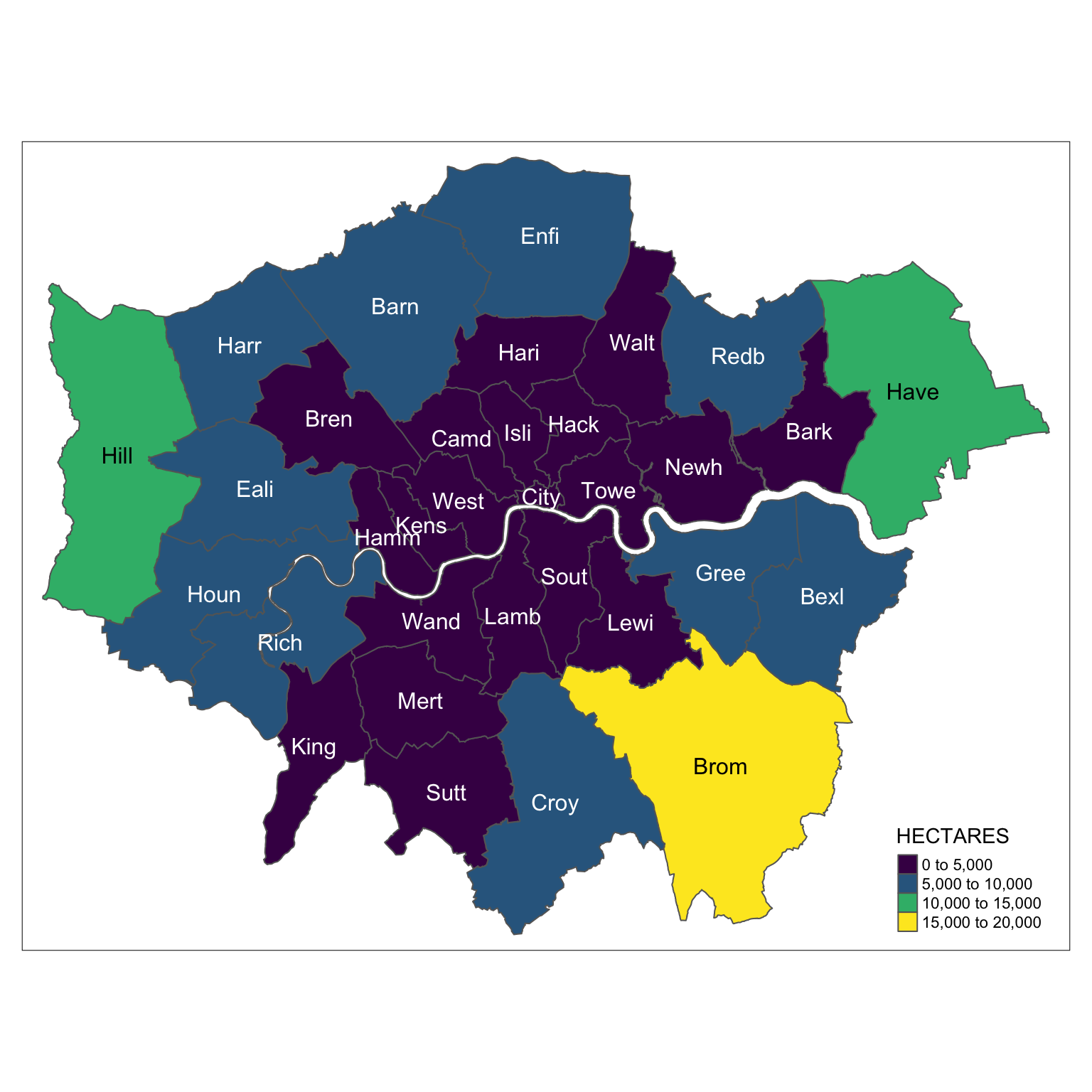So, let’s turn this into a grid to stop places like Bromley, Hillingdon and Havering from stealing our attention. First of all, we can generate a number of different grids using `seed`. Since there are many ways to dissect the outer boundary of the polygons you might want to choose an output that appeals to you. I’d recommend looking at different `seed` values and choosing the one that best matches the outline that you approve of.

The `calculate_grid` function takes in a SpatialPolygonsDataframe or sf object, a learning rate (suggestion = 0.03 to begin), a grid type `hexagonal` or `regular` and a seed value. Let’s have a look at some hexagonal grid options for the London local authorities:

``````par(mfrow = c(2, 3), mar = c(0, 0, 2, 0))
for (i in 1:6) {
new_cells <- calculate_grid(shape = original_shapes, grid_type = "hexagonal", seed = i)
plot(new_cells, main = paste("Seed", i, sep = " "))
}``````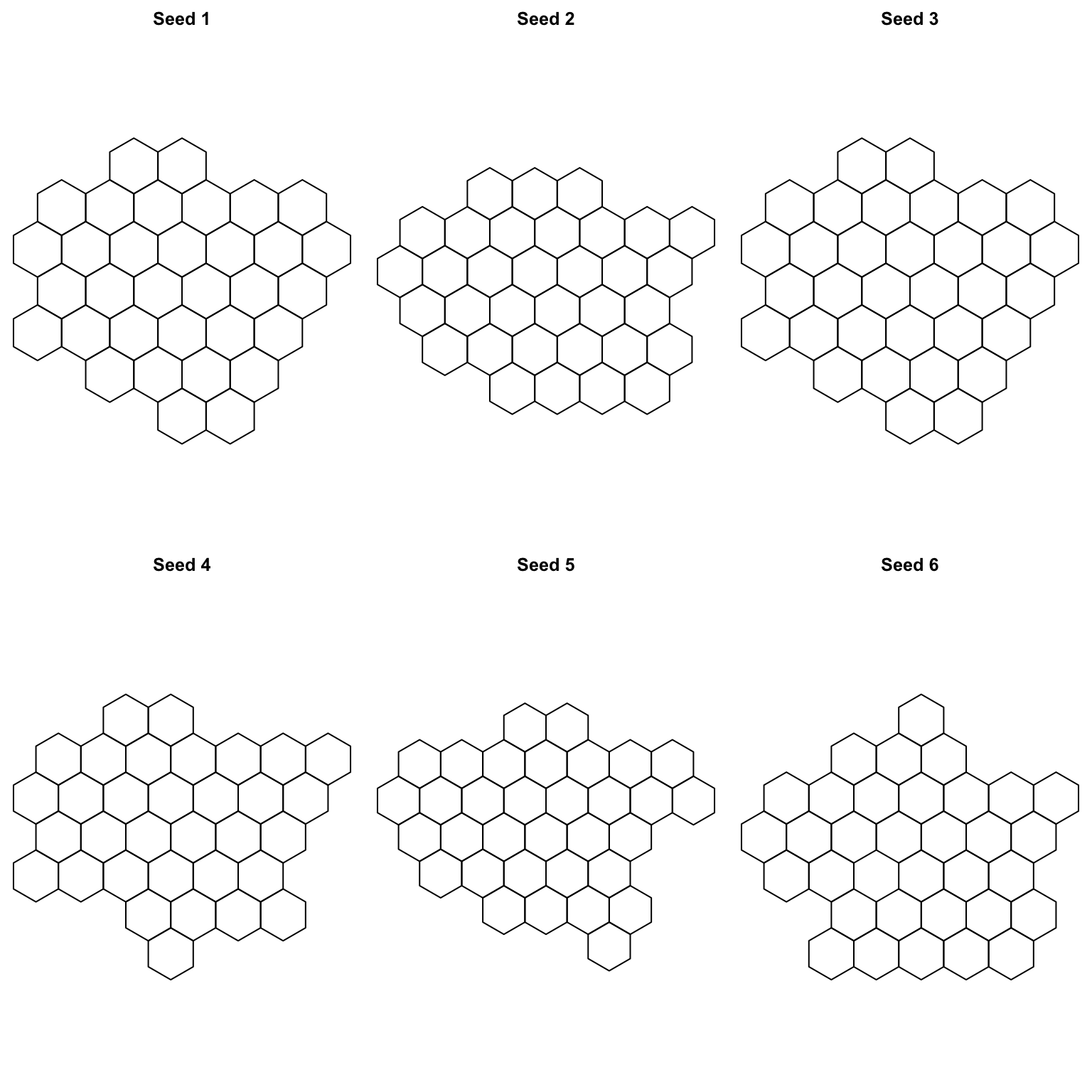Let’s also look at things with a regular grid:

``````par(mfrow = c(2, 3), mar = c(0, 0, 2, 0))
for (i in 1:6) {
new_cells <- calculate_grid(shape = original_shapes, grid_type = "regular", seed = i)
plot(new_cells, main = paste("Seed", i, sep = " "))
}``````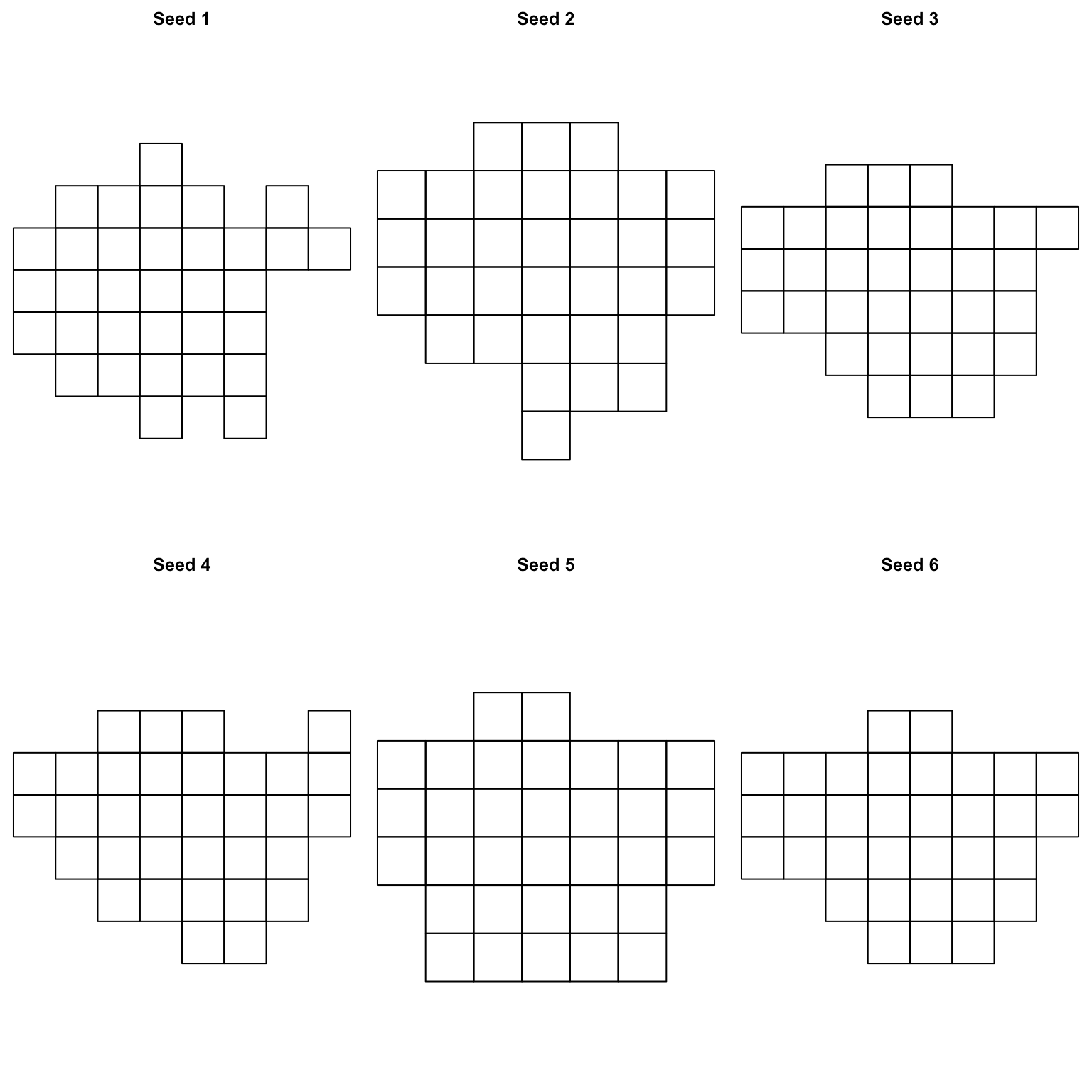As we can see there are lots of options. Now, lets choose a grid and assign our existing places to it. I happen to like the both grids that have a `seed` of 3. So I’m going to assign the polygons to those grids. Let’s do that and see what they look like compared to the original.

``````new_cells_hex <- calculate_grid(shape = original_shapes, grid_type = "hexagonal", seed = 3)
resulthex <- assign_polygons(original_shapes, new_cells_hex)

new_cells_reg <- calculate_grid(shape = original_shapes, grid_type = "regular", seed = 3)
resultreg <- assign_polygons(original_shapes, new_cells_reg)``````

Now we have an example transfer from real space to grid space - we can visualise it.

``````hexplot <- tm_shape(resulthex) +
tm_polygons("HECTARES", palette = "viridis") +
tm_text("SNAME")

regplot <- tm_shape(resultreg) +
tm_polygons("HECTARES", palette = "viridis") +
tm_text("SNAME")

tmap_arrange(rawplot, hexplot, regplot, nrow = 3)``````## Details

The package has two major functions:

1. `calculate_grid()` given your input polygons this will generate the grid as specified by your arguments:
• `shape`: the original polygons
• `learning_rate`: the rate at which the gradient descent finds the optimum cellsize to ensure that your gridded points fit within the outer boundary of the input polygons.
• `grid_type`: either `regular` for a square grid or `hexagonal` for a hexagonal grid.
• `seed`: the seed to ensure you get the same grid output.
2. `assign_polygons()`: this will assign the original polygons to their new locations on the grid generated in `calculate_grid()`. It will find the solution that minimises the sum of the total distance between the original polygon centroids and eventual gridded centroids. Arguments:
• `shape`: the original polygons
• `new_polygons`: the output (a list) from `calculate_grid()`.

## TODO

• Assignment may not always work - check the `assign_polygons()` why does it only work sometimes?
• Make it work (done I think), make it right (not yet), make it fast (not yet).
• Improve the cellsize calculation methodology.
• Get someone to answer this stack overflow question.

This is my first attempt at a package. If it doesn’t work I’d like suggestions for improvements and thanks in advance for providing them!

I welcome critique and feedback. Blog post to follow.

## Thanks

I read a lot of the work by Hadley Wickham, Jenny Bryan, Thomas Lin Pedersen, Mara Averick and Bob Rudis to name a few. But also love the R community and learn a huge amount from R Bloggers.

Extra thanks go to Ryan Hafen for making this package publishable.

# Other examples

From others:

Simon Hailstone has looked at male life expectancy in the South East region of England using the package. Thanks Simon for using!

From me:

This time using the contiguous USA. Again, I used set seed and chose some that I liked but I’d recommend you’d do the same.

``````input_file2 <- system.file("extdata", "states.json", package = "geogrid")
original_shapes2\$SNAME <- substr(original_shapes2\$NAME, 1, 4)

rawplot2 <- tm_shape(original_shapes2) +
tm_polygons("CENSUSAREA", palette = "viridis") +
tm_text("SNAME")``````

Let’s check the seeds again.

``````par(mfrow = c(2, 3), mar = c(0, 0, 2, 0))
for (i in 1:6) {
new_cells <- calculate_grid(shape = original_shapes2, grid_type = "hexagonal", seed = i)
plot(new_cells, main = paste("Seed", i, sep = " "))
}``````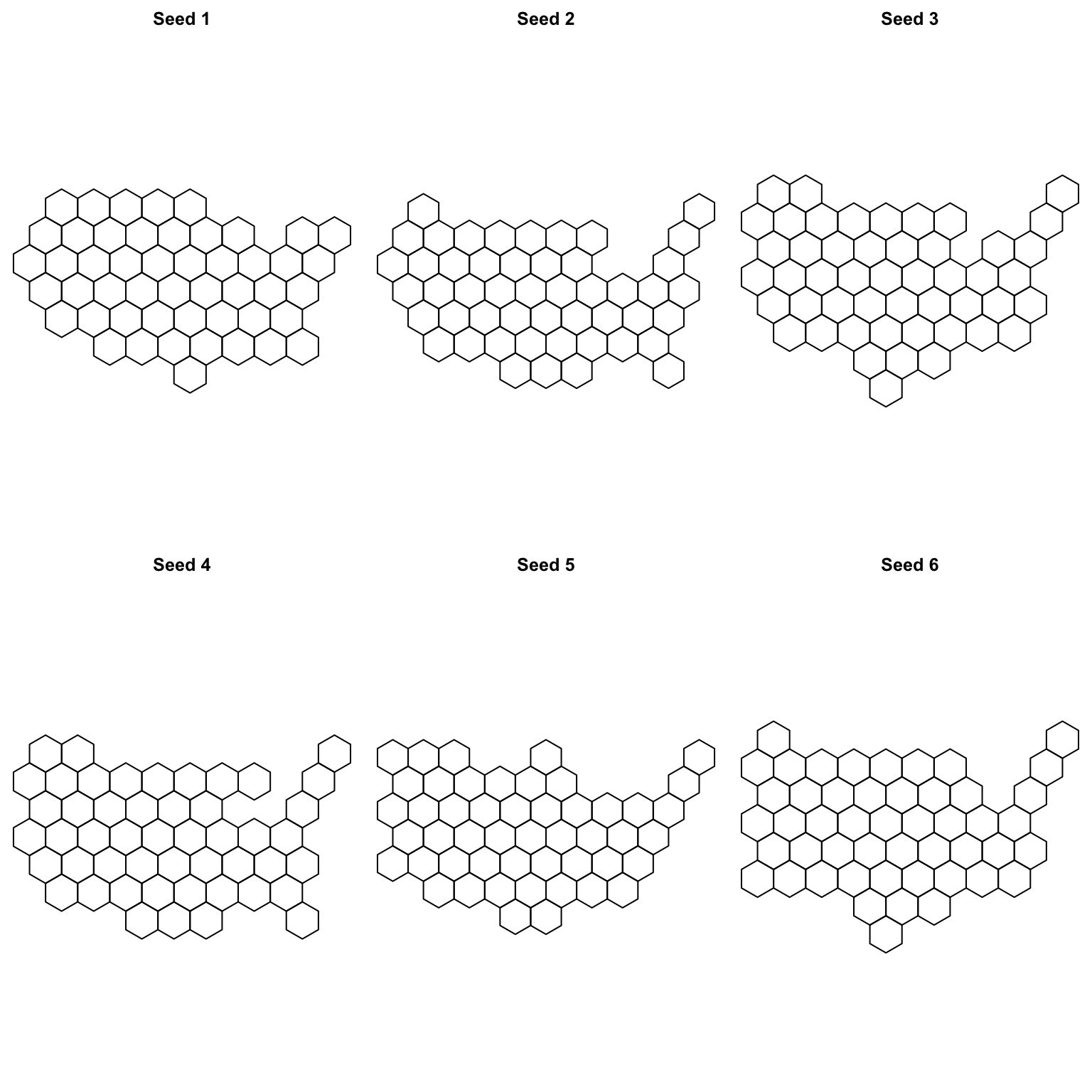``````par(mfrow = c(2, 3), mar = c(0, 0, 2, 0))
for (i in 1:6) {
new_cells <- calculate_grid(shape = original_shapes2, grid_type = "regular", seed = i)
plot(new_cells, main = paste("Seed", i, sep = " "))
}``````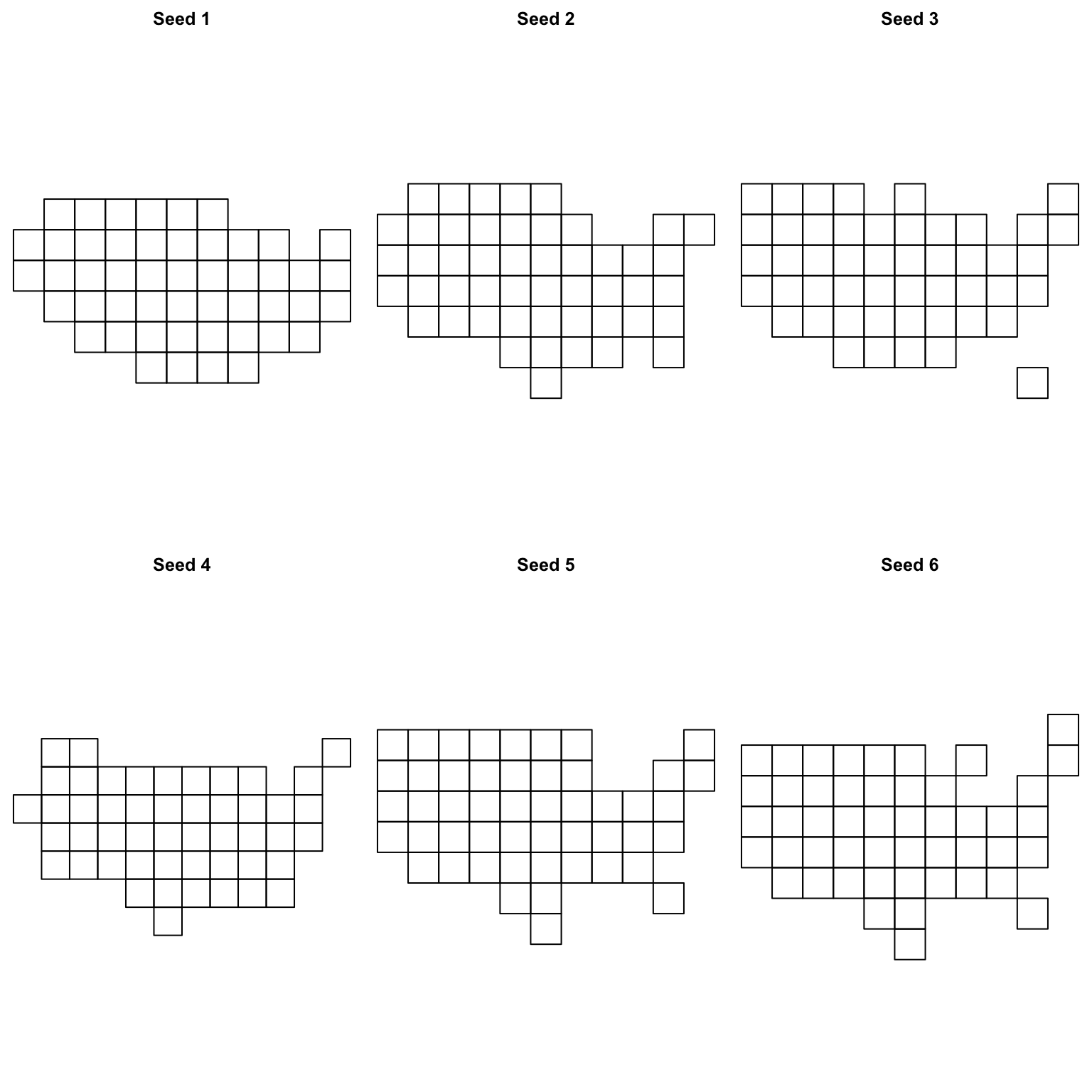Now we’ve seen some seed demo’s lets assign them…

``````new_cells_hex2 <- calculate_grid(shape = original_shapes2, grid_type = "hexagonal", seed = 6)
resulthex2 <- assign_polygons(original_shapes2, new_cells_hex2)

new_cells_reg2 <- calculate_grid(shape = original_shapes2, grid_type = "regular", seed = 4)
resultreg2 <- assign_polygons(original_shapes2, new_cells_reg2)

hexplot2 <- tm_shape(resulthex2) +
tm_polygons("CENSUSAREA", palette = "viridis") +
tm_text("SNAME")

regplot2 <- tm_shape(resultreg2) +
tm_polygons("CENSUSAREA", palette = "viridis") +
tm_text("SNAME")

tmap_arrange(rawplot2, hexplot2, regplot2, nrow = 3)``````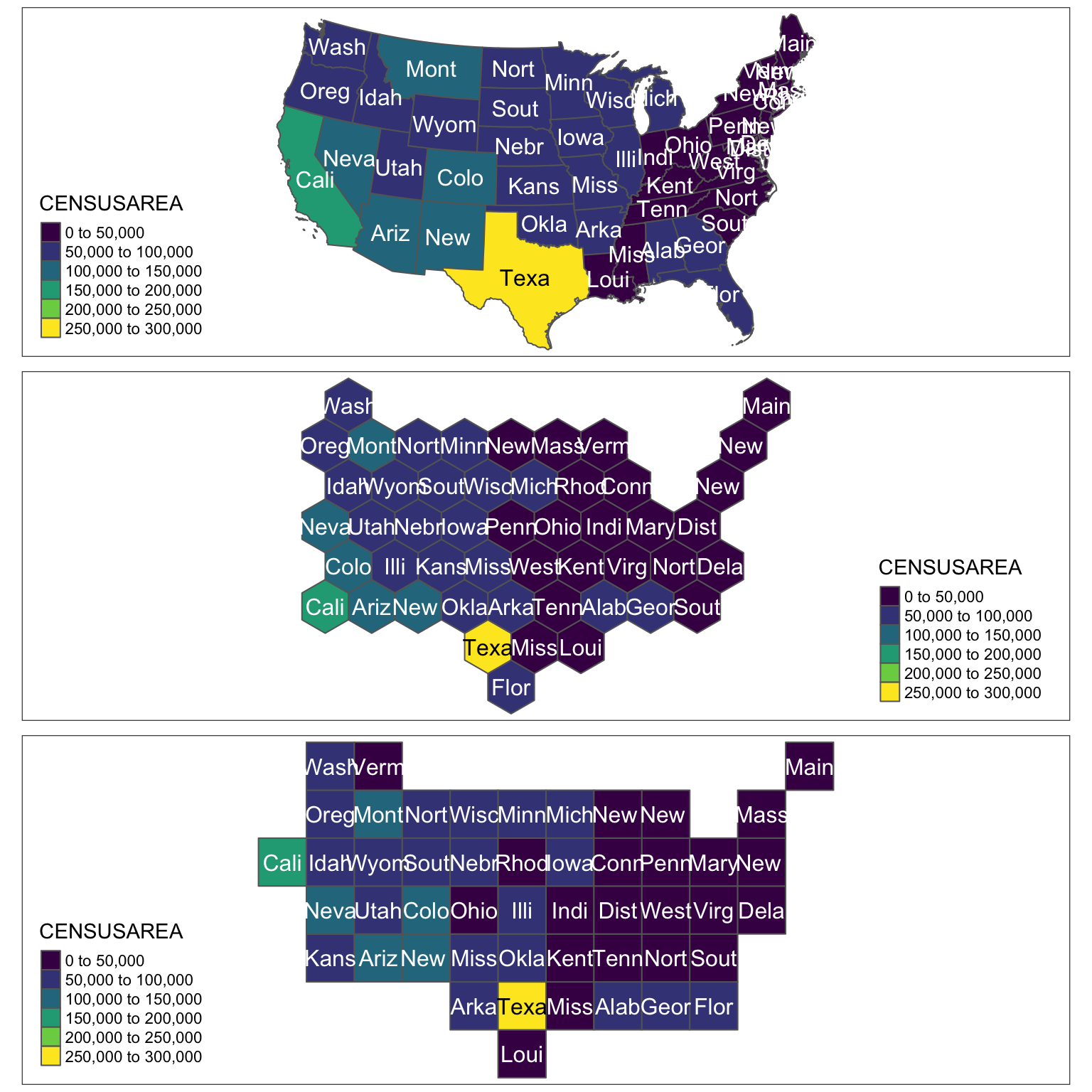Likewise, you can try the bay area…

``````input_file3 <- system.file("extdata", "bay_counties.geojson", package = "geogrid")
original_shapes3\$SNAME <- substr(original_shapes3\$county, 1, 4)

rawplot3 <- tm_shape(original_shapes3) +
tm_polygons(col = "gray25") +
tm_text("SNAME")

new_cells_hex3 <- calculate_grid(shape = original_shapes3, grid_type = "hexagonal", seed = 6)
resulthex3 <- assign_polygons(original_shapes3, new_cells_hex3)

new_cells_reg3 <- calculate_grid(shape = original_shapes3, grid_type = "regular", seed = 1)
resultreg3 <- assign_polygons(original_shapes3, new_cells_reg3)

hexplot3 <- tm_shape(resulthex3) +
tm_polygons(col = "gray25") +
tm_text("SNAME")

regplot3 <- tm_shape(resultreg3) +
tm_polygons(col = "gray25") +
tm_text("SNAME")

tmap_arrange(rawplot3, hexplot3, regplot3, nrow = 3)``````